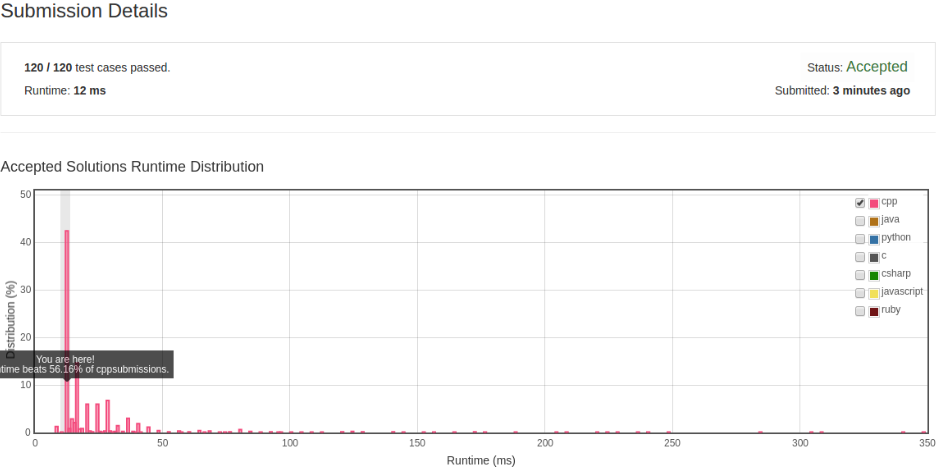# 凌云的博客

## LeetCode 算法题 16. 3Sum Closest

### 题目

Given an array S of n integers, find three integers in S such that the sum is closest to a given number, target. Return the sum of the three integers. You may assume that each input would have exactly one solution.

``````    For example, given array S = {-1 2 1 -4}, and target = 1.

The sum that is closest to the target is 2. (-1 + 2 + 1 = 2).
``````

### 解法

``````class Solution {
public:
int threeSumClosest(vector<int>& nums, int target) {
sort(nums.begin(), nums.end());
int closestDiff = INT_MAX;
for (int i = 0; i < nums.size(); i++) {
int front = i + 1;
int back = nums.size() - 1;

while (front < back) {
int diff = target - nums[i] - nums[front] - nums[back];
if (abs(closestDiff) > abs(diff)) {
closestDiff = diff;
}

if (dff > 0) {
front++;
} else if (diff < 0) {
back--;
} else {
return target;
}
}
}

return target - closestDiff;
}
};
``````